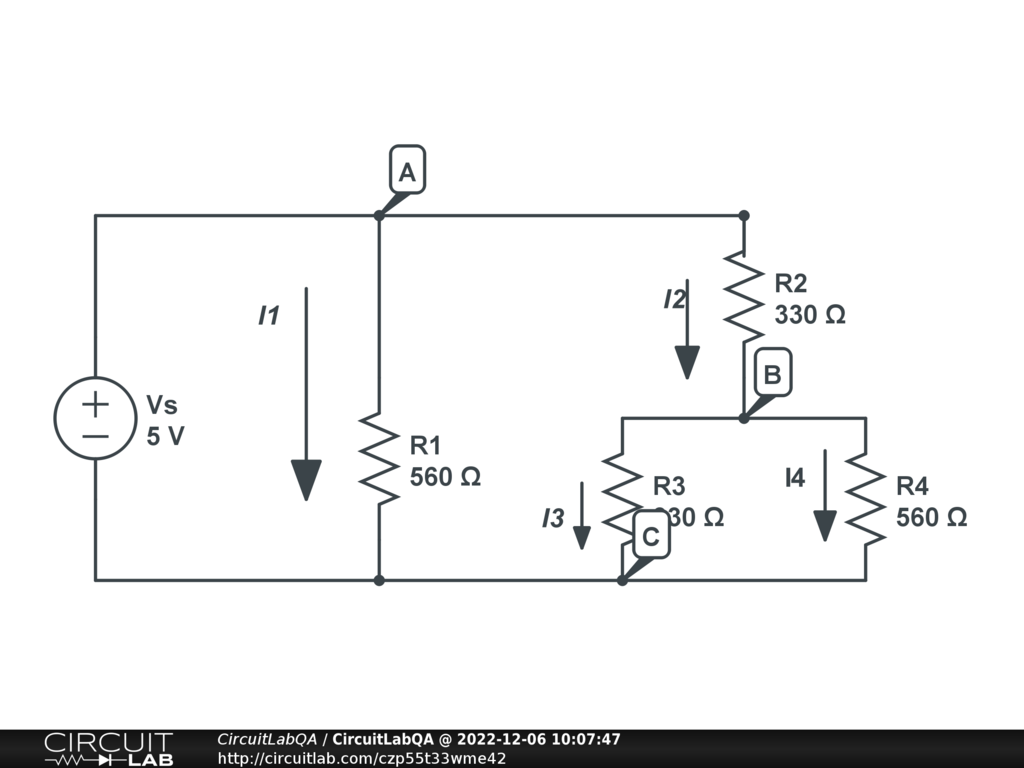## Why is my calculated current different than the book example?

 Hello, I'm teaching myself about circuits, I don't understand why my calculations are different than that of the book. The goal is to actually find I4, but I can't seem to see why I'm getting a different value for I2.Here are the book calculations: R2+3||4 = R2 + (R3R4 / R3+R4) = 330Ohms + (330Ohms)(560Ohms)/890Ohms = 538Ohms I2 = Vs/R2+3||4 = 5.0V/538Ohms = 9.29mA Here are my calculations: 1/Rt = 1/560 + 1/538 Rt = 1/0.0036444 Rt = 274.39 It = Vs/Rt It = 5.0/274.389 = 18.2mA I2 = (R1/R1+Rt)It I2 = (560/560+274.39)18.2mA = 12.21mA??? This is my first time posting, so if my formatting is wack let me know. Thank you for your time. by Lav.J December 06, 2022 You need a ground connection somewhere to get any results at all. Put a ground at the negative terminal of V6 and see if that helps by Foxx December 06, 2022 Voltage (drop) is the same between two given points whatever path you take. So, being interested in I2, we have, between points A and C: 5 = I2 * ( R2 + R3 || R4 ) Solving for I2. R1 is not involved, unless you are interested in the total current supplied by the voltage source, in which case you also have 5 = I1 * R1 Solving for R1. And total current by the voltage source: I1 + I2 You main flaw in your computation is that you made I2 dependent of R1, which is not. by vanderghast December 07, 2022 When using the voltage divider formula, instead of using the total resistance of the right-side branch, I used the total resistance of the circuit. "I2 = (R1/R1+Rt)It I2 = (560/560+274.39)18.2mA = 12.21mA??? " Should have been this -> I2 = (560/560+538)18.2 = 9.29mA Thanks for the replies though by Lav.J December 08, 2022 R1 and R2 are not in series and so, a voltage divider notion does not apply. Make R3 = R4 = 0, for simplicity. Rtotal = R1 R2 / ( R1 + R2) Itotal = 5 / Rt all that is ok, but now: I2 = (R1/(R1 + Rtotal)) Itotal is not. Indeed, I2 = 5 / R2 (always with R3=R4=0) as well as I1 = 5 / R1 Your formula for I2 leads to $$I_2 = \frac{ 5 R_1} {R_1 + \frac{R_1 R_2}{R_1+R_2}} \frac{R_1+R_2}{R_1 R_2}$$ Which "simplifies" to $$\frac{5 (R_1 + R_2) ^2 }{R_1 R_2 (R_1 + 2 R_2)}$$ (unless I made a mistake, which is possible), but clearly weird. A voltage divider is valid for two resistors in series (not in parallel), with a negligible difference in the current through each of them. That is not the case at all, here. by vanderghast December 08, 2022

CircuitLab's Q&A site is a FREE questions and answers forum for electronics and electrical engineering students, hobbyists, and professionals.

We encourage you to use our built-in schematic & simulation software to add more detail to your questions and answers.

Acceptable Questions:

• Concept or theory questions
• Practical engineering questions
• “Homework” questions
• Software/hardware intersection
• Best practices
• Design choices & component selection
• Troubleshooting

Unacceptable Questions:

• Non-English language content
• Non-question discussion
• Non-electronics questions
• Vendor-specific topics
• Pure software questions
• CircuitLab software support

Please respect that there are both seasoned experts and total newbies here: please be nice, be constructive, and be specific!